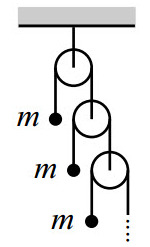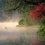# Infinite Atwood (pulley) system - what's wrong with this?Each block has mass $m$. We are finding acceleration of topmost block.

Assume the tension $T$ acting on topmost block and acceleration $a$ in upward direction.

Then tension on second block is $\frac{T}{2}$. If we see the block w.r.t the pulley that it is connected to, then apply psuedo force $ma$ in upward direction (as the pulley it is connected to is moving in downward with $a$), then the system observed is same as the above, I.e acceleration will be same.

Hence $T-mg=ma\\ \frac{T}{2}+ma\text{ (psuedo force) }-mg=ma$

We obtain $a=g$ but the answer should be ...(this is a question on brilliant and I can't share answer)Note by Megh Parikh
6 years, 11 months ago

This discussion board is a place to discuss our Daily Challenges and the math and science related to those challenges. Explanations are more than just a solution — they should explain the steps and thinking strategies that you used to obtain the solution. Comments should further the discussion of math and science.

When posting on Brilliant:

• Use the emojis to react to an explanation, whether you're congratulating a job well done , or just really confused .
• Ask specific questions about the challenge or the steps in somebody's explanation. Well-posed questions can add a lot to the discussion, but posting "I don't understand!" doesn't help anyone.
• Try to contribute something new to the discussion, whether it is an extension, generalization or other idea related to the challenge.

MarkdownAppears as
*italics* or _italics_ italics
**bold** or __bold__ bold
- bulleted- list
• bulleted
• list
1. numbered2. list
1. numbered
2. list
Note: you must add a full line of space before and after lists for them to show up correctly
paragraph 1paragraph 2

paragraph 1

paragraph 2

[example link](https://brilliant.org)example link
> This is a quote
This is a quote
    # I indented these lines
# 4 spaces, and now they show
# up as a code block.

print "hello world"
# I indented these lines
# 4 spaces, and now they show
# up as a code block.

print "hello world"
MathAppears as
Remember to wrap math in $$ ... $$ or $ ... $ to ensure proper formatting.
2 \times 3 $2 \times 3$
2^{34} $2^{34}$
a_{i-1} $a_{i-1}$
\frac{2}{3} $\frac{2}{3}$
\sqrt{2} $\sqrt{2}$
\sum_{i=1}^3 $\sum_{i=1}^3$
\sin \theta $\sin \theta$
\boxed{123} $\boxed{123}$

Sort by:

All the blocks will be relative to each other as all the blocks are not stable, and thus the acceleration of each block will be different and you took them as same. just find the relation between the accelerations of different blocks and find a sequence and similarly you will find that acceleration of topmost block is g/2. ,try by taking a suitable observable position.

- 6 years, 11 months ago

You misunderstood me.

I am seeing block2 wrt pulley2. Then pulley2 is at rest and it also has a system similar to pulley one. The acceleration of block2 is $a$ wrt pulley2

- 6 years, 11 months ago

See the mistake which you did while solving this senario....

https://brilliant.org/community-problem/infinite-atwood-machine/

- 6 years, 11 months ago

Are you able to access solutions. I am waiting long but the solutions are just not coming on mobile. Will have to try on computer. Thanks anyway

There is also another such question which i am resharing so that you may answer it.

- 6 years, 11 months ago

Its the same problem which u asked..here only you have to assume that g=9.8 m/ss in place of 10m/ss

- 6 years, 11 months ago

No see my comment in that question on your solution.

I have no problem with 10 & 9.8

- 6 years, 11 months ago

- 6 years, 11 months ago

Why is the acceleration of the 2nd pulley wrt 2nd block $a$?

- 6 years, 11 months ago

Why do you think it is the same system?

- 6 years, 11 months ago